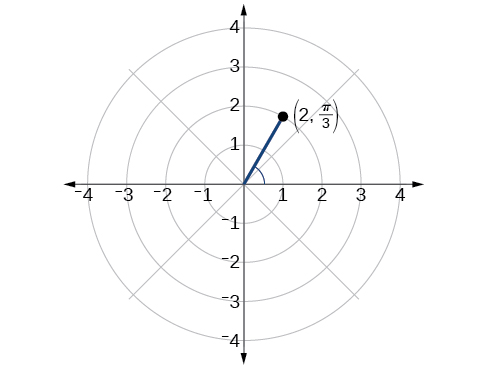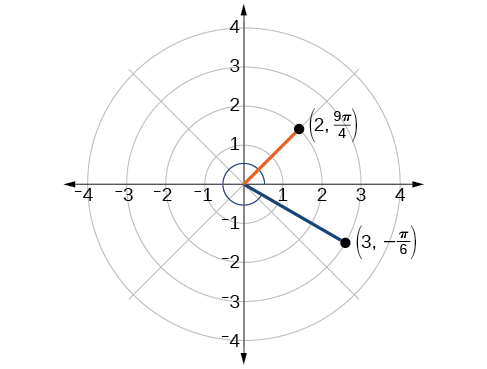# 10.3 Polar coordinates

 Page 1 / 8
In this section, you will:
• Plot points using polar coordinates.
• Convert from polar coordinates to rectangular coordinates.
• Convert from rectangular coordinates to polar coordinates.
• Transform equations between polar and rectangular forms.
• Identify and graph polar equations by converting to rectangular equations.

Over 12 kilometers from port, a sailboat encounters rough weather and is blown off course by a 16-knot wind (see [link] ). How can the sailor indicate his location to the Coast Guard? In this section, we will investigate a method of representing location that is different from a standard coordinate grid.

## Plotting points using polar coordinates

When we think about plotting points in the plane, we usually think of rectangular coordinates $\text{\hspace{0.17em}}\left(x,y\right)\text{\hspace{0.17em}}$ in the Cartesian coordinate plane. However, there are other ways of writing a coordinate pair and other types of grid systems. In this section, we introduce to polar coordinates    , which are points labeled $\text{\hspace{0.17em}}\left(r,\theta \right)\text{\hspace{0.17em}}$ and plotted on a polar grid. The polar grid is represented as a series of concentric circles radiating out from the pole    , or the origin of the coordinate plane.

The polar grid is scaled as the unit circle with the positive x- axis now viewed as the polar axis    and the origin as the pole. The first coordinate $\text{\hspace{0.17em}}r\text{\hspace{0.17em}}$ is the radius or length of the directed line segment from the pole. The angle $\text{\hspace{0.17em}}\theta ,$ measured in radians, indicates the direction of $\text{\hspace{0.17em}}r.\text{\hspace{0.17em}}$ We move counterclockwise from the polar axis by an angle of $\text{\hspace{0.17em}}\theta ,$ and measure a directed line segment the length of $\text{\hspace{0.17em}}r\text{\hspace{0.17em}}$ in the direction of $\text{\hspace{0.17em}}\theta .\text{\hspace{0.17em}}$ Even though we measure $\text{\hspace{0.17em}}\theta \text{\hspace{0.17em}}$ first and then $\text{\hspace{0.17em}}r,$ the polar point is written with the r -coordinate first. For example, to plot the point $\text{\hspace{0.17em}}\left(2,\frac{\pi }{4}\right),$ we would move $\text{\hspace{0.17em}}\frac{\pi }{4}\text{\hspace{0.17em}}$ units in the counterclockwise direction and then a length of 2 from the pole. This point is plotted on the grid in [link] .

## Plotting a point on the polar grid

Plot the point $\text{\hspace{0.17em}}\left(3,\frac{\pi }{2}\right)\text{\hspace{0.17em}}$ on the polar grid.

The angle $\text{\hspace{0.17em}}\frac{\pi }{2}\text{\hspace{0.17em}}$ is found by sweeping in a counterclockwise direction 90° from the polar axis. The point is located at a length of 3 units from the pole in the $\text{\hspace{0.17em}}\frac{\pi }{2}\text{\hspace{0.17em}}$ direction, as shown in [link] .

Plot the point $\text{\hspace{0.17em}}\left(2,\text{\hspace{0.17em}}\frac{\pi }{3}\right)\text{\hspace{0.17em}}$ in the polar grid .## Plotting a point in the polar coordinate system with a negative component

Plot the point $\text{\hspace{0.17em}}\left(-2,\text{\hspace{0.17em}}\frac{\pi }{6}\right)\text{\hspace{0.17em}}$ on the polar grid.

We know that $\text{\hspace{0.17em}}\frac{\pi }{6}\text{\hspace{0.17em}}$ is located in the first quadrant. However, $\text{\hspace{0.17em}}r=-2.\text{\hspace{0.17em}}$ We can approach plotting a point with a negative $\text{\hspace{0.17em}}r\text{\hspace{0.17em}}$ in two ways:

1. Plot the point $\text{\hspace{0.17em}}\left(2,\frac{\pi }{6}\right)\text{\hspace{0.17em}}$ by moving $\text{\hspace{0.17em}}\frac{\pi }{6}\text{\hspace{0.17em}}$ in the counterclockwise direction and extending a directed line segment 2 units into the first quadrant. Then retrace the directed line segment back through the pole, and continue 2 units into the third quadrant;
2. Move $\text{\hspace{0.17em}}\frac{\pi }{6}\text{\hspace{0.17em}}$ in the counterclockwise direction, and draw the directed line segment from the pole 2 units in the negative direction, into the third quadrant.

See [link] (a). Compare this to the graph of the polar coordinate $\text{\hspace{0.17em}}\left(2,\frac{\pi }{6}\right)\text{\hspace{0.17em}}$ shown in [link] (b).

Plot the points $\text{\hspace{0.17em}}\left(3,-\frac{\pi }{6}\right)$ and $\text{\hspace{0.17em}}\left(2,\frac{9\pi }{4}\right)\text{\hspace{0.17em}}$ on the same polar grid.## Converting from polar coordinates to rectangular coordinates

When given a set of polar coordinates    , we may need to convert them to rectangular coordinates . To do so, we can recall the relationships that exist among the variables $\text{\hspace{0.17em}}x,\text{\hspace{0.17em}}y,\text{\hspace{0.17em}}r,\text{\hspace{0.17em}}$ and $\text{\hspace{0.17em}}\theta .$

#### Questions & Answers

x exposant 4 + 4 x exposant 3 + 8 exposant 2 + 4 x + 1 = 0
x exposent4+4x exposent3+8x exposent2+4x+1=0
HERVE
How can I solve for a domain and a codomains in a given function?
ranges
EDWIN
Thank you I mean range sir.
Oliver
proof for set theory
don't you know?
Inkoom
find to nearest one decimal place of centimeter the length of an arc of circle of radius length 12.5cm and subtending of centeral angle 1.6rad
factoring polynomial
find general solution of the Tanx=-1/root3,secx=2/root3
find general solution of the following equation
Nani
the value of 2 sin square 60 Cos 60
0.75
Lynne
0.75
Inkoom
when can I use sin, cos tan in a giving question
depending on the question
Nicholas
I am a carpenter and I have to cut and assemble a conventional roof line for a new home. The dimensions are: width 30'6" length 40'6". I want a 6 and 12 pitch. The roof is a full hip construction. Give me the L,W and height of rafters for the hip, hip jacks also the length of common jacks.
John
I want to learn the calculations
where can I get indices
I need matrices
Nasasira
hi
Raihany
Hi
Solomon
need help
Raihany
maybe provide us videos
Nasasira
Raihany
Hello
Cromwell
a
Amie
What do you mean by a
Cromwell
nothing. I accidentally press it
Amie
you guys know any app with matrices?
Khay
Ok
Cromwell
Solve the x? x=18+(24-3)=72
x-39=72 x=111
Suraj
Solve the formula for the indicated variable P=b+4a+2c, for b
Need help with this question please
b=-4ac-2c+P
Denisse
b=p-4a-2c
Suddhen
b= p - 4a - 2c
Snr
p=2(2a+C)+b
Suraj
b=p-2(2a+c)
Tapiwa
P=4a+b+2C
COLEMAN
b=P-4a-2c
COLEMAN
like Deadra, show me the step by step order of operation to alive for b
John
A laser rangefinder is locked on a comet approaching Earth. The distance g(x), in kilometers, of the comet after x days, for x in the interval 0 to 30 days, is given by g(x)=250,000csc(π30x). Graph g(x) on the interval [0, 35]. Evaluate g(5)  and interpret the information. What is the minimum distance between the comet and Earth? When does this occur? To which constant in the equation does this correspond? Find and discuss the meaning of any vertical asymptotes.
The sequence is {1,-1,1-1.....} hasByByBy Sam Luong